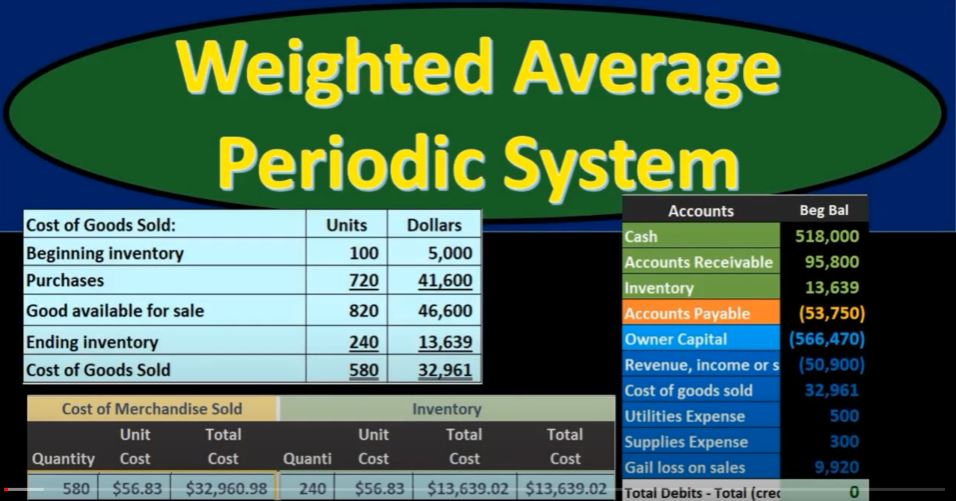## Weighted Average Periodic System 46

In this presentation, we will delve into the weighted average inventory method using a periodic system. We’ll also explore the weighted average method in contrast to the first-in, first-out (FIFO) or last-in, first-out (LIFO) methods, as well as the differences between periodic and perpetual inventory systems. To better grasp these concepts, we’ll work through an illustrative worksheet.

The Inventory Flow Systems:

Before we dive into the weighted average method, it’s essential to understand that there are various inventory flow systems, including:

1. First-In, First-Out (FIFO)
2. Last-In, First-Out (LIFO)
3. Weighted Average Method

These methods can be implemented under both perpetual and periodic inventory systems, making it vital to comprehend their implications.

Setting Up the Worksheet:

Let’s start by setting up our worksheet to address inventory-related questions. This standard format simplifies the process, regardless of the specific inventory method we use.

Our worksheet comprises three primary components:

1. Purchases: This section tracks the quantity, unit cost, and total cost of inventory purchases.
2. Cost of Merchandise Sold: This area records the quantity, unit cost, and total cost of inventory items sold.
3. Inventory or Ending Inventory: Here, we monitor the quantity, unit cost, and total cost of the remaining inventory.

Beginning Inventory:

We’ll kick off with the beginning inventory, which represents the inventory on hand at the start of the accounting period. For instance, let’s assume we had 100 units of inventory valued at \$50 each, totaling \$5,000.

Purchases:

Next, we make inventory purchases. In March, we acquired 400 units at a cost of \$55 each, resulting in a total purchase cost of \$22,000. To calculate the weighted average cost, we need to determine the weighted average unit cost as we proceed.

Calculating the Weighted Average:

Calculating the weighted average unit cost is crucial. Instead of taking a simple average, which assumes equal weight for all costs, we must consider the quantity of each purchase. To do this:

1. Sum the total dollar amount of purchases (in this case, \$22,000).
2. Sum the total quantity of purchases (in this case, 100 units from the beginning inventory plus 400 units purchased equals 500 units).
3. Divide the total dollar amount by the total quantity to calculate the weighted average unit cost.

In this case, the weighted average unit cost is approximately \$44 (\$22,000 / 500 units).

Subsequent Purchases:

We continue purchasing inventory throughout the accounting period. For instance, we acquire 120 units at \$60 each and another 200 units at \$62 each. We record these purchases in the “Purchases” section of our worksheet.

Cost of Goods Sold:

Now, we move on to the cost of goods sold (COGS). In a periodic system, we do not record COGS at the time of each sale as in a perpetual system. Instead, we calculate COGS at the end of the period, based on physical inventory counts.

COGS Calculation:

To calculate COGS, we use the following formula:

• Beginning Inventory + Purchases = Goods Available for Sale
• Goods Available for Sale – Ending Inventory = COGS

In our case, we had a beginning inventory of \$5,000, made purchases totaling \$41,600, and determined our ending inventory to be \$13,006.39. The difference between goods available for sale and ending inventory gives us our COGS, which is \$32,960.61.

Final Journal Entry:

Finally, we record a journal entry to adjust the inventory and recognize the COGS. This is the adjustment that only occurs at the end of the accounting period in a periodic system.

• Debit Inventory: Reducing it by \$32,960.61
• Credit COGS: Increasing it by \$32,960.61

Conclusion:

In a periodic system, the weighted average method allows us to calculate COGS at the end of the period by considering the weighted average unit cost of inventory. While this method simplifies day-to-day accounting, it requires a final adjustment to accurately reflect financial statements and income.

Understanding these inventory flow methods and their applications is crucial for making informed financial decisions in accounting and business management.